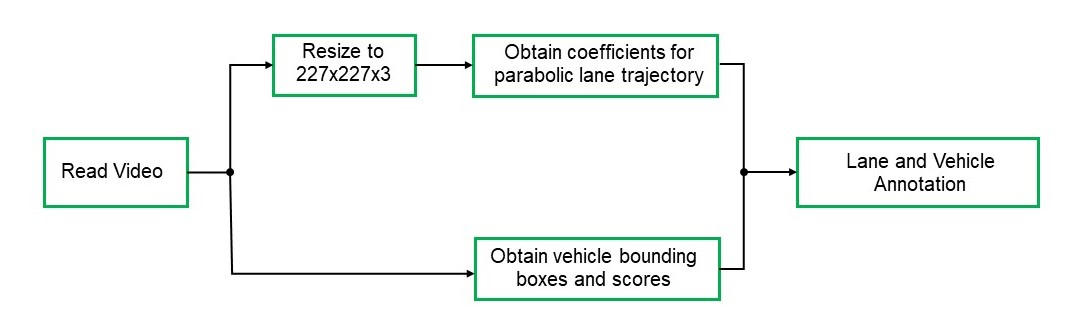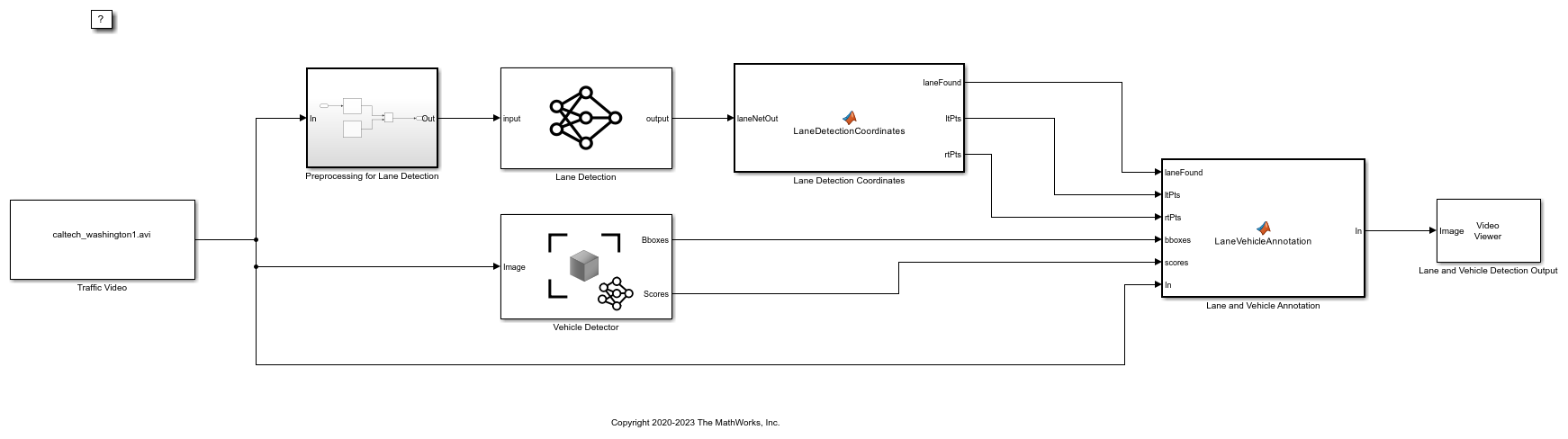# Lane and Vehicle Detection in Simulink Using Deep Learning

This example shows how to use deep convolutional neural networks inside a Simulink® model to perform lane and vehicle detection. This example takes the frames from a traffic video as an input, outputs two lane boundaries that correspond to the left and right lanes of the ego vehicle, and detects vehicles in the frame.

This example uses the pretrained lane detection network from the Lane Detection Optimized with GPU Coder example of the GPU Coder Toolbox™. For more information, see Lane Detection Optimized with GPU Coder (GPU Coder).

This example also uses the pretrained vehicle detection network from the Object Detection Using YOLO v2 Deep Learning example of the Computer Vision toolbox™. For more information, see Object Detection Using YOLO v2 Deep Learning (Computer Vision Toolbox).

### Algorithmic Workflow

The block diagram for the algorithmic workflow of the Simulink model is shown.### Get Pretrained Lane and Vehicle Detection Networks

The `getVehicleDetectionAndLaneDetectionNetworks` function downloads the `trainedLaneNet.mat` and `yolov2ResNet50VehicleExample.mat` files if they are not already present.

```getVehicleDetectionAndLaneDetectionNetworks() ```
```Downloading pretrained lane detection network (143 MB)... Downloading pretrained vehicle detection network (98 MB)... ```

```if ~exist('./caltech_washington1.avi', 'file') url = 'https://www.mathworks.com/supportfiles/gpucoder/media/caltech_washington1.avi'; websave('caltech_washington1.avi', url); end ```

### Lane and Vehicle Detection Simulink Model

The Simulink model for performing lane and vehicle detection on the traffic video is shown. When the model runs, the `Video Viewer` block displays the traffic video with lane and vehicle annotations.

```open_system('laneAndVehicleDetectionMDL'); ```### Lane Detection

For lane detection, the traffic video is preprocessed by resizing each frame of the video to 227-by-227-by-3 and then scaled by a factor of 255. The preprocessed frames are then input to the `trainedLaneNet.mat` network loaded in the `Predict` block from the Deep Learning Toolbox™. This network takes an image as an input and outputs two lane boundaries that correspond to the left and right lanes of the ego vehicle. Each lane boundary is represented by the parabolic equation:

``

Here y is the lateral offset and x is the longitudinal distance from the vehicle. The network outputs the three parameters a, b, and c per lane. The network architecture is similar to `AlexNet` except that the last few layers are replaced by a smaller fully connected layer and regression output layer. The `Lane Detection Coordinates` MATLAB function block defines a function `lane_detection_coordinates` that takes the output from the predict block and outputs three parameters; `laneFound`, `ltPts`, and `rtPts`. Thresholding is used to determine if both left and right lane boundaries are both found. If both are found, `laneFound` is set to be true and the trajectories of the boundaries are calculated and stored in `ltPts` and `rtPts` respectively.

```type lane_detection_coordinates ```
```function [laneFound,ltPts,rtPts] = lane_detection_coordinates(laneNetOut) % Copyright 2020 The MathWorks, Inc. persistent laneCoeffMeans; if isempty(laneCoeffMeans) laneCoeffMeans = [-0.0002 0.0002 1.4740 -0.0002 0.0045 -1.3787]; end persistent laneCoeffStds; if isempty(laneCoeffStds) laneCoeffStds = [0.0030 0.0766 0.6313 0.0026 0.0736 0.9846]; end params = laneNetOut .* laneCoeffStds + laneCoeffMeans; isRightLaneFound = abs(params(6)) > 0.5; %c should be more than 0.5 for it to be a right lane isLeftLaneFound = abs(params(3)) > 0.5; persistent vehicleXPoints; if isempty(vehicleXPoints) vehicleXPoints = 3:30; %meters, ahead of the sensor end ltPts = coder.nullcopy(zeros(28,2,'single')); rtPts = coder.nullcopy(zeros(28,2,'single')); if isRightLaneFound && isLeftLaneFound rtBoundary = params(4:6); rt_y = computeBoundaryModel(rtBoundary, vehicleXPoints); ltBoundary = params(1:3); lt_y = computeBoundaryModel(ltBoundary, vehicleXPoints); % Visualize lane boundaries of the ego vehicle tform = get_tformToImage; % map vehicle to image coordinates ltPts = tform.transformPointsInverse([vehicleXPoints', lt_y']); rtPts = tform.transformPointsInverse([vehicleXPoints', rt_y']); laneFound = true; else laneFound = false; end end ```

### Vehicle Detection

This example uses a YOLO v2 based network for vehicle detection. A YOLO v2 object detection network is composed of two subnetworks: a feature extraction network followed by a detection network. This pretrained network uses a `ResNet-50` for feature extraction. The detection sub-network is a small CNN compared to the feature extraction network and is composed of a few convolutional layers and layers specific to YOLO v2.

The Simulink model performs vehicle detection inside the `MATLAB Function` block `Vehicle Detection YOLOv2`. This function block defines a function `vehicle_detection_yolo_v2` that loads the pretrained YOLO v2 detector. This network takes an image as input and outputs the bounding box coordinates along with the confidence scores for vehicles in the image.

```type vehicle_detection_yolo_v2 ```
```function [bboxes,scores] = vehicle_detection_yolo_v2(In) % Copyright 2020 The MathWorks, Inc. persistent yolodetector; if isempty(yolodetector) yolodetector = coder.loadDeepLearningNetwork('yolov2ResNet50VehicleExample.mat'); end [bboxes,scores,~] = yolodetector.detect(In, 'threshold', .2); end ```

### Annotation of Vehicle Bounding Boxes and Lane Trajectory in Traffic Video

The `Lane and Vehicle Annotation` MATLAB function block defines a function `lane_vehicle_annotation` which annotates the vehicle bounding boxes along with the confidence scores. If `laneFound` is true, then the left and right lane boundaries stored in `ltPts` and `rtPts` are overlayed on the traffic video.

```type lane_vehicle_annotation ```
```function In = lane_vehicle_annotation(laneFound, ltPts, rtPts, bboxes, scores, In) % Copyright 2020 The MathWorks, Inc. if ~isempty(bboxes) In = insertObjectAnnotation(In, 'rectangle', bboxes, scores); end pts = coder.nullcopy(zeros(28, 4, 'single')); if laneFound prevpt = [ltPts(1,1) ltPts(1,2)]; for k = 2:1:28 pts(k,1:4) = [prevpt ltPts(k,1) ltPts(k,2)]; prevpt = [ltPts(k,1) ltPts(k,2)]; end In = insertShape(In, 'Line', pts, 'LineWidth', 2); prevpt = [rtPts(1,1) rtPts(1,2)]; for k = 2:1:28 pts(k,1:4) = [prevpt rtPts(k,1) rtPts(k,2)]; prevpt = [rtPts(k,1) rtPts(k,2)]; end In = insertShape(In, 'Line', pts, 'LineWidth', 2); In = insertMarker(In, ltPts); In = insertMarker(In, rtPts); end end ```

### Run the Simulation

To verify the lane and vehicle detection algorithms and display the lane trajectories, vehicle bounding boxes and scores for the traffic video loaded in the Simulink model, run the simulation.

```set_param('laneAndVehicleDetectionMDL', 'SimulationMode', 'Normal'); sim('laneAndVehicleDetectionMDL'); ```
```Warning: Saturate on overflow detected. In MATLAB Function '<a href="matlab:Stateflow.Debug.Runtime.open_object('laneAndVehicleDetectionMDL/Lane and Vehicle Annotation', 0);">laneAndVehicleDetectionMDL/Lane and Vehicle Annotation</a>': function In = LaneVehicleAnnotation(laneFound, ltPts, rtPts, bboxes, scores, In) Suggested Actions: • Suppress future instances of this diagnostic from this source. - <a href="matlab:Simulink.SuppressedDiagnostic({'laneAndVehicleDetectionMDL/Lane and Vehicle Annotation'},'Stateflow:Runtime:DataSaturateError');">Suppress</a> ```### Code Generation

With GPU Coder™, you can accelerate the execution of model on NVIDIA® GPUs and generate CUDA® code for model. See the Code Generation for a Deep Learning Simulink Model that Performs Lane and Vehicle Detection (GPU Coder) for more details.

### Cleanup

```close_system('laneAndVehicleDetectionMDL/Lane and Vehicle Detection Output'); close_system('laneAndVehicleDetectionMDL'); ```